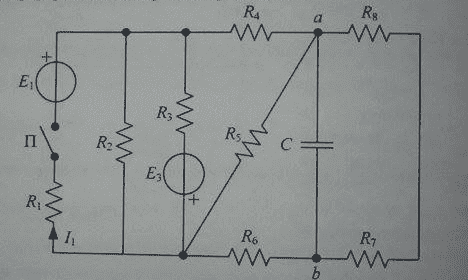# Calculating net change of capacitor charge in a circuit

• Engineering

## Homework Statement

This problem apparently has a simple solution if you are good with circuit theorems but no matter what i try or how i combine them, it's just impossible. Given a circuit on the picture, and circuit parameters E3=12V,R1=1kΩ,R2=30Ω,R3=150Ω,R6=200Ω, if it is known that current I1 = 60 mA when the switch (P) is closed, calculate the net change of capacitor charge between switch states ( switch is first closed, and then opened - there is also a time delay between switch changes so we are only interested in stationary states).
EDIT: Capacitor charge for when switch is closed is Q=-2 µC
EDIT: Thanks gneill, I made a mistake. E3 is known, not E1## Homework Equations

Ohm's law - U = R*I
Current devider
Thevenein and Norton theorem
Superposition theorem
Node potential method
Mesh current method ( i doub't this is useful in this example )[/B]

## The Attempt at a Solution

I did try but i just can't get anything useful. I don't even have capacitor capacitance so i can't calculate voltage (Uab). Millman's theorem came to mind ( for when the switch is open, we can calculate voltage U1 in at the end of edge with E3, and then compensate that edge with an ideal voltage generator with EMS equal to U1. From here, since there is no current flow trough the capacitor, we have a single generator connected to a resistor network but we don't know 4 out of 5 resistors' resistances) but it seems like a dead end. As for the situation with switch closed, that's even worse. No matter what i do, I just can;t "reach the capacitor" ...

Last edited:

gneill
Mentor
Is E3 also an unknown quantity?

I updated the question. E3 is known, not E1. I apologize.

gneill
Mentor
So to confirm, unknown component values are:
E1
R4
R5
R7
R8
C

Yes, that is correct.